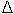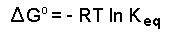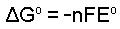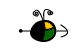Free Energy Changes

The free energy change of a chemical process under standard state conditions,Go, can be determined four different ways:

• From Free Energies of Formation
• From Enthalpy Changes and Entropy Changes
• From Equilibrium Constants
• From Cell Potentials
• Using Free Energies of Formation to Determine Standard State Free Energy Changes

If we know the standard free energy changes of formation,Gof, of each species in a change we can determine the standard state free energy change,Go, for the change using the following equation:Top

Using Enthalpy Changes and Entropy Changes to Determine Standard State Free Energy Changes

If we know the enthalpy change,Ho, and the entropy change,So, for a chemical process, we can determine the standard state free energy change,Go, for the process using the following equation:In this equation T is the temperature on the Kelvin scale. In introductory courses we make the assumption thatHo andSo, do not change as the temperature changes.

Top

Using Equilibrium Constants to Determine Standard State Free Energy Changes

If we know the equilibrium constant, Keq, for a chemical change (or if we can determine the equilibrium constant), we can calculate the standard state free energy change,Go, for the reaction using the equation:In this equation

• R = 8.314 J mol-1 K-1 or 0.008314 kJ mol-1 K-1.
• T is the temperature on the Kelvin scale.
• Keq is the equilibrium constant at the temperature T.

Top

Using Cell Potentials to Determine Standard State Free Energy Changes

If we know the standard state cell potential, Eo, for an electrochemical cell (or if we can determine the standard state cell potential), we can calculate the standard state free energy change,Go, for the cell reaction using the equation:In this equation

• n is the number of moles of electrons exchanged in the cell reaction.
• F is 96.485 kJ volt-1 mole-1 (the "Faraday").
• Eo is the cell potential under standard state conditions.

Top